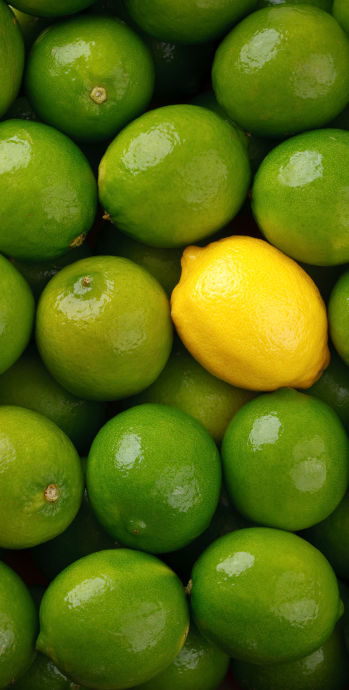#### You may also likeMake a set of numbers that use all the digits from 1 to 9, once and once only. Add them up. The result is divisible by 9. Add each of the digits in the new number. What is their sum? Now try some other possibilities for yourself!### Counting Factors

Is there an efficient way to work out how many factors a large number has?### Repeaters

Choose any 3 digits and make a 6 digit number by repeating the 3 digits in the same order (e.g. 594594). Explain why whatever digits you choose the number will always be divisible by 7, 11 and 13.

# Differences

##### Age 11 to 14Challenge Level

Differences printable worksheetChoose any three whole numbers, find the differences between them all, and find the product of the differences.

For example, if your three whole numbers are $7$, $4$ and $12$, the differences are:

$12 - 7 = 5$
$12 - 4 = 8$
$7 - 4 = 3$

The product of the differences is
$3\times 5\times 8 = 120$

Try a few examples.

What do you notice?
Can you explain what you've noticed?

Now choose any four whole numbers, find the differences between them all, and find the product of the differences.

For example, if your four whole numbers are $7$, $4$, $12$ and $6$, the differences are:

$7-4 = 3$
$12-7 = 5$
$7-6 = 1$
$12-4 = 8$
$6-4 = 2$
$12-6 = 6$

The product of the differences is $3\times 5\times 1\times 8\times 2\times 6 = 1440$

Try a few examples.

What do you notice?
Can you explain what you've noticed?